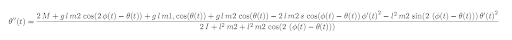## Tuesday, May 06, 2008

### Onager simulation

I wanted to make a general method/program to create a model of a (simple) physical system (connected rigid bodies in all basic shapes). The basic analysis is done by hand, but scripts/programs in Matlab and Mathematica do all the mathematical and computational work. I applied it to an onager. I want to build, at least, a mini onager based on this model. This is the onager concept:What I modelled is a stiff beam with a weightless stiff rope/pipe connected at the end. The projectile at the end of this rope is modelled as a point mass. A constant moment on the first beam was added to model the force that the rope/spring exerts on the beam.

The input variables for the model are: mass of beam, mass of projectile, length of beam and length of rope.

While it may look like a simple problem, the mathematical description of the motion (equations of motion) of this system is rather complex. This is one of the two equations of motion:Here is the resulting slow motion animation of the motion, with velocity vectors and velocity (in m/s) added to the animation. It simulates a mini onager (30cm arm) with a large, but realistic, moment on the arm shooting a mass of 10g:

And here are some animations of the motion when the moment on the arm is zero (no force on the rope). This is a chaotic system where very small changes in initial conditions result in a very different motion. This also means that small computation errors (for example the limited number of digits used in a computer or the error of the numerical integration) result in a different motion. It should behave natural though.

very large mass

equal mass beam and weight

animation of system on earth.
same system but on the moon.
I don't really have an easy way to verify my model other than by visual inspection.